# How to print Boolean values in PythonLast updated: Feb 20, 2023
3 min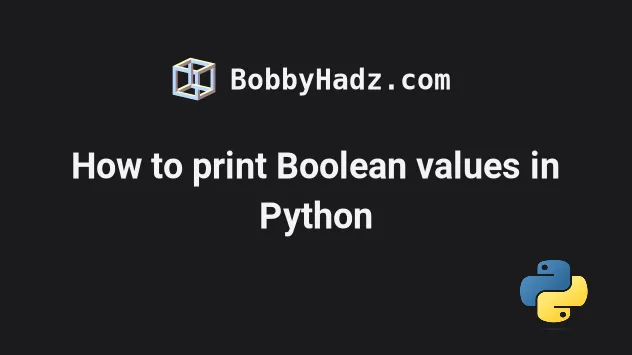## #Print boolean values in Python

Use the `print()` function to print a boolean value in Python, e.g. `print(my_bool)`.

If the value is not of type boolean, use the `bool()` class to convert it to a boolean and print the result, e.g. `bool(0)`.

main.py
```Copied!```my_bool = True

# ✅ print boolean value
print(my_bool)  # 👉️ True

# ✅ print boolean value in a string
print(f'is subscribed: {my_bool}')  # 👉️ is subscribed: True

# ✅ print integer representation of boolean value
print(int(True))  # 👉️ 1
print(int(False))  # 👉️ 0

# ✅ print boolean value converted to lowercase
print(f'{my_bool}'.lower())  # 👉️ true

# ✅ Convert a value to a boolean
print(bool('hello'))  # 👉️ True
print(bool(0))  # 👉️ False
``````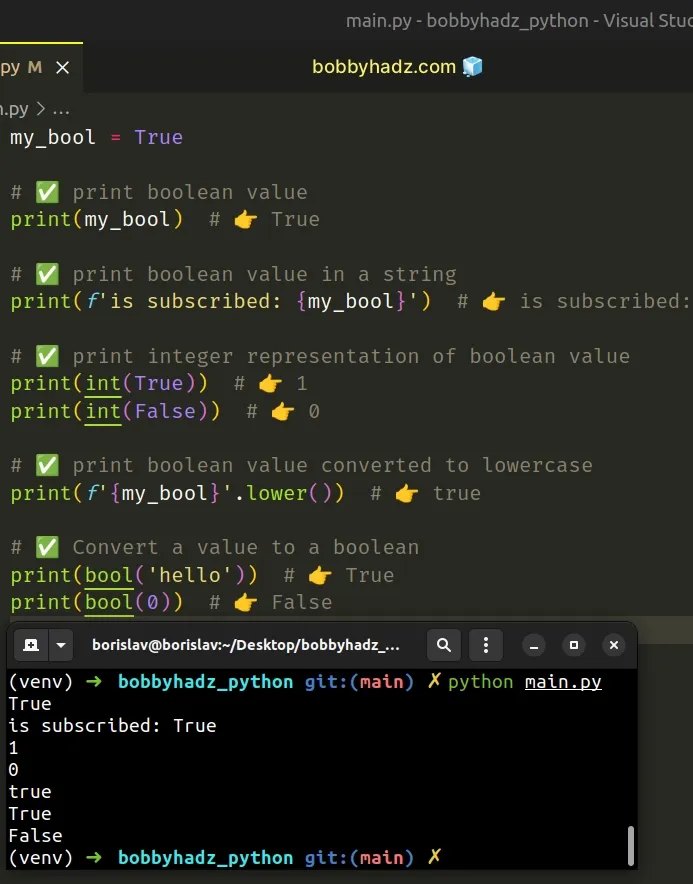We used the `print()` function to print boolean values.

The print function takes one or more objects and prints them to `sys.stdout`.

If you have a boolean value, you can pass it directly to the `print()` function to print it.

main.py
```Copied!```my_bool = True

print(my_bool)  # 👉️ True

print(False) # 👉️ False
``````
Note that the `print()` function returns `None`, so don't try to store the result of calling `print` in a variable.
main.py
```Copied!```my_bool = True

# ⛔️ BAD (print always returns None)
result = print(f'is subscribed: {my_bool}')

print(result)  # 👉️ None
``````

Instead, store the value in a variable and pass the variable to the `print()` function.

main.py
```Copied!```my_bool = True
result = f'is subscribed: {my_bool}'

print(result)  # 👉️ is subscribed: True
``````

If you aren't sure what type a variable stores, use the built-in `type()` class.

main.py
```Copied!```my_bool = False
print(type(my_bool))  # 👉️ <class 'bool'>
print(isinstance(my_bool, bool))  # 👉️ True

my_str = 'hello'
print(type(my_str))  # 👉️ <class 'str'>
print(isinstance(my_str, str))  # 👉️ True
``````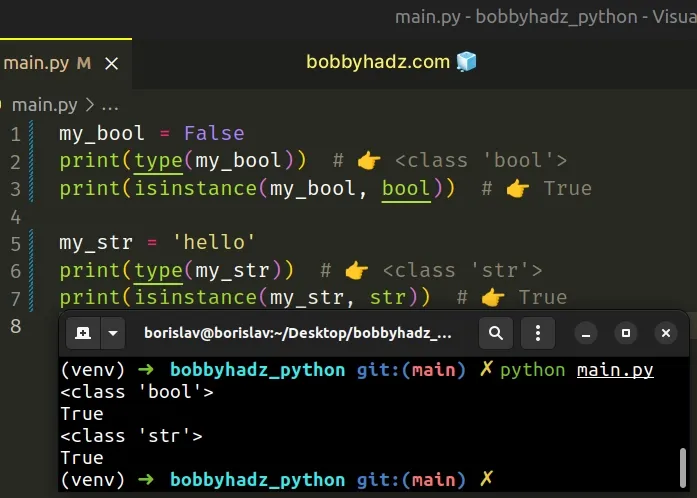The type class returns the type of an object.

The isinstance function returns `True` if the passed-in object is an instance or a subclass of the passed in class.

## #Converting a value to a boolean and printing the result

If you need to convert a value to a boolean and print the result, use the bool() class.

main.py
```Copied!```print(bool('hello'))  # 👉️ True

print(bool(0))  # 👉️ False
``````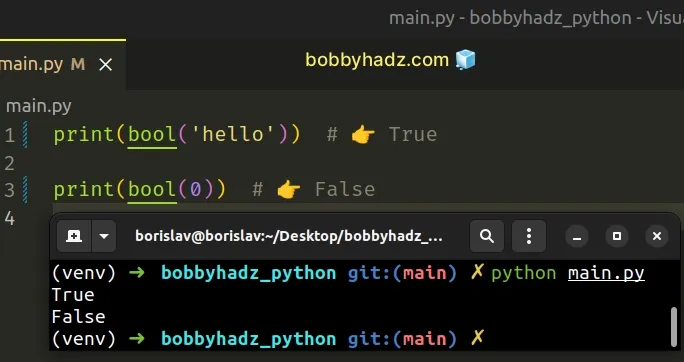The bool class converts truthy values to `True` and falsy values to `False`.

All values that are not truthy are considered falsy. The falsy values in Python are:

• constants defined to be falsy: `None` and `False`.
• `0` (zero) of any numeric type.
• empty sequences and collections: `""` (empty string), `()` (empty tuple), `[]` (empty list), `{}` (empty dictionary), `set()` (empty set), `range(0)` (empty range).

## #Printing a boolean value in a String

If you need to print a boolean value or a variable storing a boolean value in a string, use a formatted string literal.

main.py
```Copied!```my_bool = True

# ✅ print boolean value in a string
print(f'is subscribed: {my_bool}')  # 👉️ is subscribed: True
``````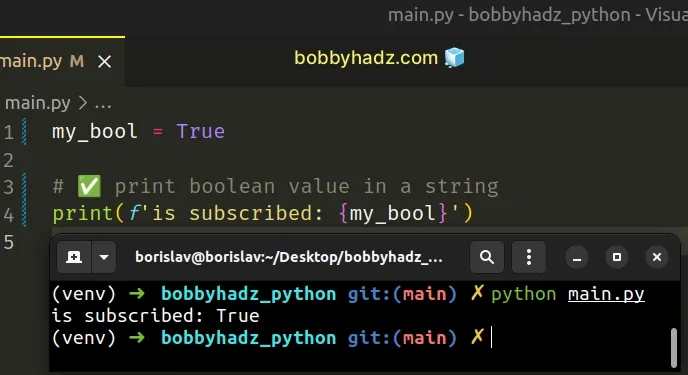Formatted string literals (f-strings) let us include expressions inside of a string by prefixing the string with `f`.

Make sure to wrap expressions in curly braces - `{expression}`.

## #Passing multiple, comma-separated arguments to print()

Alternatively, you can pass multiple, comma-separated arguments to the `print()` function.

main.py
```Copied!```# 👇️ is subscribed: True
print('is subscribed:', True)

# 👇️ is subscribed:True
print('is subscribed:', True, sep='')
``````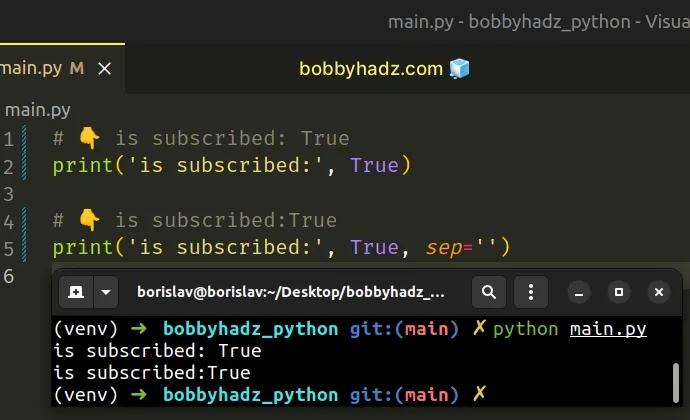By default, when you pass multiple, comma-separated arguments to the `print()` function, they get separated by a space.

You can set the sep keyword argument to an empty string to remove the separator.

If you need to convert a boolean value to its integer representation, use the `int()` class.

main.py
```Copied!```print(int(True))  # 👉️ 1
print(int(False))  # 👉️ 0
``````

The int class returns an integer object constructed from the provided number or string argument.

Conversely, if you need to convert an integer to a boolean value, use the `bool()` class.

main.py
```Copied!```print(bool(1))  # 👉️ True
print(bool(0))  # 👉️ False
``````

The bool() class takes a value and converts it to a boolean (True or False). If the provided value is falsy or omitted, then `bool` returns `False`, otherwise it returns `True`.